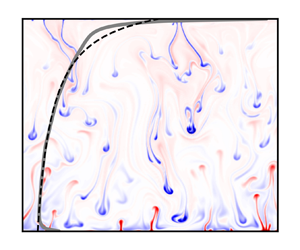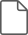Hostname: page-component-7d8f8d645b-9fg92 Total loading time: 0 Render date: 2023-05-28T06:51:06.498Z Has data issue: false Feature Flags: { "useRatesEcommerce": true } hasContentIssue false

## AbstractIn the quest to understand the basic universal features of compressible convection, one would like to disentangle genuine consequences of compression from spatial variations of transport properties. For instance, one may choose to consider a fluid with uniform dynamic viscosity, but, then, compressible effects will generate a density gradient and consequently the kinematic viscosity will not be uniform. In the present work, we consider a very peculiar equation of state, whereby entropy is solely dependent on density, so that a nearly isentropic fluid domain is nearly isochoric. Within this class of equations of state, there is a thermal adiabatic gradient and a key property of compressible convection is still present, namely its capacity to viscously dissipate a large fraction of the thermal energy involved, of the order of the well-named dissipation number. In the anelastic approximation, under the assumption of an infinite Prandtl number, the number of governing parameters can be brought down to two, the Rayleigh number and the dissipation number. This framework is proposed as a playground for compressible convection, an opportunity to extend the vast corpus of theoretical analyses on the Oberbeck–Boussinesq equations regarding stability, bifurcations or the determination of upper bounds for the turbulent heat transfer. Here, in a two-dimensional geometry, we concentrate on the structure of numerical solutions. For all Rayleigh numbers, a change in the vertical temperature profile is observed in the range of dissipation number between$0$and less than$0.4$, associated with the weakening of ascending plumes. For larger dissipation numbers, the heat flux dependence on this number is found to be well predicted by Malkus's model of critical layers. For dissipation numbers of order unity, and large Rayleigh numbers, dissipation becomes related to the entropy heat flux at each depth, so that the vertical dissipation profile can be predicted, and so does the total ratio of dissipation to convective heat flux.

## JFM classification

Type
JFM Papers
Information
Journal of Fluid Mechanics , 10 June 2022 , A9

## Access options

Get access to the full version of this content by using one of the access options below. (Log in options will check for institutional or personal access. Content may require purchase if you do not have access.)

## References

#### REFERENCES

Alboussière, T. & Ricard, Y. 2013 Reflections on dissipation associated with thermal convection. J. Fluid Mech. 725, 14697645.CrossRefGoogle Scholar
Alboussière, T. & Ricard, Y. 2017 Rayleigh–Bénard stability and the validity of quasi-Boussinesq or quasi-anelastic liquid approximations. J. Fluid Mech. 817, 264305.CrossRefGoogle Scholar
Anders, E.H., Lecoanet, D. & Brown, B. 2019 Entropy rain: dilution and compression of thermals in stratified domains. Astrophys. J. 884, 65.CrossRefGoogle Scholar
Anufriev, A.P., Jones, C.A. & Soward, A.M. 2005 The Boussinesq and anelastic liquid approximations for convection in the Earth's core. Phys. Earth Planet. Inter. 152, 163190.CrossRefGoogle Scholar
Backus, G.E. 1975 Gross thermodynamics of heat engines in deep interior of Earth. Proc. Natl Acad. Sci. USA 72 (4), 15551558.CrossRefGoogle ScholarPubMed
Bazarov, I.P. 1989 Thermodynamique. Ed. MIR.Google Scholar
Bénard, H. 1901 Les Tourbillons cellulaires dans une nappe liquide propageant de la chaleur par convection, en régime permanent. Thèse de la Faculté des Sciences de Paris.Google Scholar
Bormann, A.S. 2001 The onset of convection in the Rayleigh–Bénard problem for compressible fluids. Contin. Mech. Thermodyn. 13, 923.CrossRefGoogle Scholar
Boussinesq, J. 1903 Théorie analytique de la chaleur, tome 2, pp. 157161. Gauthier-Villars.Google Scholar
Braginsky, S.I. & Roberts, P.H. 1995 Equations governing convection in Earth's core and the geodynamo. Geophys. Astrophys. Fluid Dyn. 79, 197.CrossRefGoogle Scholar
Burns, K.J., Vasil, G.M., Oishi, J.S., Lecoanet, D. & Brown, B.P. 2020 Dedalus: a flexible framework for numerical simulations with spectral methods. Phys. Rev. Res. 2, 023068.CrossRefGoogle Scholar
Busse, F.H. 1967 The stability of finite amplitude cellular convection and its relation to an extremum principle. J. Fluid Mech. 30 (4), 625649.CrossRefGoogle Scholar
Carnot, S. 1824 Réflexions sur la puissance motrice du feu. Bachelier Libraire.Google Scholar
Chan, K.L. & Sofia, S. 1989 Turbulent compressible convection in a deep atmosphere. IV. Results of three-dimensional computations. Astrophys. J. 336, 10221040.CrossRefGoogle Scholar
Curbelo, J., Duarte, L., Alboussière, T., Dubuffet, F., Labrosse, S. & Ricard, Y. 2019 Numerical solutions of compressible convection with an infinite Prandtl number: comparison of the anelastic and anelastic liquid models with the exact equations. J. Fluid Mech. 873, 646687.CrossRefGoogle Scholar
Currie, L.K. & Browning, M.K. 2017 The magnitude of viscous dissipation in strongly stratified two-dimensional convection. Astrophys. J. Lett. 845, L17.CrossRefGoogle Scholar
Durran, D.R. 1989 Improving the anelastic approximation. J. Atmos. Sci. 46 (11), 14531461.2.0.CO;2>CrossRefGoogle Scholar
Featherstone, N.A. & Hindman, B.W. 2016 The spectral amplitude of stellar convection and its scaling in the high-Rayleigh-number regime. Astrophys. J. 818 (1), 32.CrossRefGoogle Scholar
Fröhlich, J., Laure, P. & Peyret, R. 1992 Large departure from Boussinesq approximation in the Rayleigh–Bénard problem. Phys. Fluids 4 (7), 13551372.CrossRefGoogle Scholar
Fuentes, J.R. & Cumming, A. 2020 Penetration of a cooling convective layer into a stably-stratified composition gradient: entrainment at low Prandtl number. Phys. Rev. Fluids 5, 124501.CrossRefGoogle Scholar
Garaud, P. 2020 Horizontal shear instabilities at low Prandtl number. Astrophys. J. 901 (2), 146.CrossRefGoogle Scholar
Gebhart, B. 1962 Effects of viscous dissipation in natural convection. J. Fluid Mech. 14 (2), 225232.CrossRefGoogle Scholar
Gilbert, G.T. 1991 Positive definite matrices and Sylvester's criterion. Am. Math. Mon. 98 (1), 4446.CrossRefGoogle Scholar
Giterman, M.S. & Shteinberg, V.A. 1970 Criteria of occurrence of free convection in a compressible viscous heat-conducting fluid. Z. Angew. Math. Mech. 34 (2), 305311.CrossRefGoogle Scholar
Goluskin, D., Johnston, H., Flierl, G.R. & Spiegel, E.A. 2014 Convectively driven shear and decreased heat flux. J. Fluid Mech. 759, 360385.CrossRefGoogle Scholar
Grandi, D. & Passerini, A. 2021 Approximation à la Oberbeck–Boussinesq for fluids with pressure-induced stratified density. Geophys. Astrophys. Fluid Dyn. 115 (4), 412435.CrossRefGoogle Scholar
Grossmann, S. & Lohse, D. 2000 Scaling in thermal convection: a unifying theory. J. Fluid Mech. 407, 2756.CrossRefGoogle Scholar
Hewitt, J.M., McKenzie, D.P. & Weiss, N.O. 1975 Dissipative heating in convective flows. J. Fluid Mech. 68 (4), 721738.CrossRefGoogle Scholar
Horn, S., Shishkina, O. & Wagner, C. 2013 On non-Oberbeck–Boussinesq effects in three-dimensional Rayleigh–Bénard convection in glycerol. J. Fluid Mech. 724, 175202.CrossRefGoogle Scholar
Howard, L.N. 1963 Heat transport by turbulent convection. J. Fluid Mech. 17 (3), 405432.CrossRefGoogle Scholar
Howard, L.N. 1966 Convection at high Rayleigh number. In Applied Mechanics (ed. H. Görtler). Springer.CrossRefGoogle Scholar
Jeffreys, H. 1930 The instability of a compressible fluid heated below. Proc. Camb. Phil. Soc. 26 (2), 170172.CrossRefGoogle Scholar
Jones, C.A., Mizerski, K. & Kessar, M. 2022 Fully developed anelastic convection with no-slip boundaries. J. Fluid Mech. 930, A13.CrossRefGoogle Scholar
Käpylä, P.J., Viviani, M., Käpylä, M.J., Brandenburg, A. & Spada, F. 2019 Effects of a subadiabatic layer on convection and dynamos in spherical wedge simulations. Geophys. Astrophys. Fluid Dyn. 113 (1–2), 149183.CrossRefGoogle Scholar
King, S.D., Lee, C., van Keken, P.E., Leng, W., Zhong, S., Tan, E., Tosi, N. & Kameyama, M.C. 2010 A community benchmark for 2-D Cartesian compressible convection in the Earth's mantle. Geophys. J. Intl 180, 7387.CrossRefGoogle Scholar
Lantz, S.R. & Fan, Y. 1999 Anelastic magnetohydrodynamic equations for modeling solar and stellar convection zones. Astrophys. J. 121, 247264.CrossRefGoogle Scholar
Lecoanet, D., Brown, B.P., Zweibel, E.G., Burns, K.J., Oishi, J.S. & Vasil, G.M. 2014 Conduction in low Mach number flows. I. Linear and weakly nonlinear regimes. Astrophys. J. 797 (2), 94.CrossRefGoogle Scholar
Lipps, F.B. 1990 On the anelastic approximation for deep convection. J. Atmos. Sci. 47 (14), 17941798.2.0.CO;2>CrossRefGoogle Scholar
Malkus, W.V.R. 1954 The heat transport and spectrum of thermal turbulence. Proc. R. Soc. A 225, 196212.Google Scholar
Oberbeck, A. 1879 Über die Wärmeleitung des Flüssigkeiten bei Berücksichtigung des Strömungen infolge von Temperaturdifferenzen. Ann. Phys. Chem. 7, 271292.CrossRefGoogle Scholar
Ogura, Y. & Phillips, N.A. 1961 Scale analysis of deep and shallow convection in the atmosphere. J. Atmos. Sci. 19, 173179.2.0.CO;2>CrossRefGoogle Scholar
Paolucci, S. & Chenoweth, D.R. 1987 Departures from the Boussinesq approximation in laminar Bénard convection. Phys. Fluids 30 (5), 15611564.CrossRefGoogle Scholar
Rayleigh, J.W.S. 1916 On convection currents in a horizontal layer of fluid, when the higher temperature is on the under side. Lond. Edinb. Dub. Philos. Mag. J. Sci. 32 (192), 529546.CrossRefGoogle Scholar
Ricard, Y. 2015 Physics of mantle convection. In Treatise on Geophysics (ed. D. Bercovici & G. Schubert), vol. 7. Cambridge University Press.CrossRefGoogle Scholar
Ricard, Y., Alboussière, T., Labrosse, S., Curbelo, J. & Dubuffet, F. 2022 Fully compressible convection for planetary mantles. Geophys. J. Intl ggac102.CrossRefGoogle Scholar
Roche, P.-E., Castaing, B., Chabaud, B. & Hébral, B. 2002 Prandtl and Rayleigh numbers dependences in Rayleigh–Bénard convection. Europhys. Lett. 58 (5), 693698.CrossRefGoogle Scholar
Schaeffer, N., Jault, D., Nataf, H.-C. & Fournier, A. 2017 Turbulent geodynamo simulations: a leap towards Earth's core. Geophys. J. Intl 211, 129.CrossRefGoogle Scholar
Schwarzschild, K. 1906 Über das Gleichgewicht des Sonnenatmosphäre. Nachr. Kgl. Ges. Wiss. Gött. Math. Phys. Klasse 1, 4153.Google Scholar
Scott, N.H. 2001 Thermoelasticity with thermomechanical constraints. Intl J. Non-Linear Mech. 36, 549564.CrossRefGoogle Scholar
Sotin, L. & Labrosse, S. 1999 Three-dimensional thermal convection in an iso-viscous, infinite Prandtl number fluid heated from within and from below: applications to the transfer of heat through planetary mantles. Phys. Earth Planet. Int. 112, 171190.CrossRefGoogle Scholar
Spiegel, E.A. 1965 Convective instability in a compressible atmosphere. I. Astrophys. J. 141 (3), 10681090.CrossRefGoogle Scholar
Spiegel, E.A. & Veronis, G. 1971 On the boussinesq approximation for a compressible fluid. Astrophys. J. 131, 442447.CrossRefGoogle Scholar
Tilgner, A. 2011 Convection in an ideal gas at high Rayleigh numbers. Phys. Rev. E 84 (2), 026323.CrossRefGoogle Scholar
Vasil, G.M., Lecoanet, D., Brown, B.P., Wood, T. & Zweibel, E.G. 2013 Energy conservation and gravity waves in sound-proof treatments of stellar interiors. II. Lagrangian constrained analysis. Astrophys. J. 773, 169.CrossRefGoogle Scholar
Viallet, M., Meakin, C., Arnett, D. & Mocák, M. 2013 Turbulent convection in stellar interiors . III. Mean-field analysis and stratification effects. Astrophys. J. 769, 1.CrossRefGoogle Scholar
Wang, X. 2004 Infinite Prandtl number limit of Rayleigh–Bénard convection. Commun. Pure Appl. Maths 57 (10), 12651282.CrossRefGoogle Scholar### Alboussière et al. supplementary material

Supplementary data files

File 13 KB